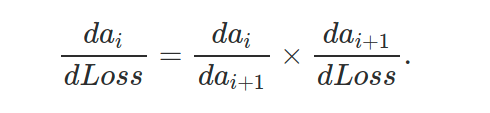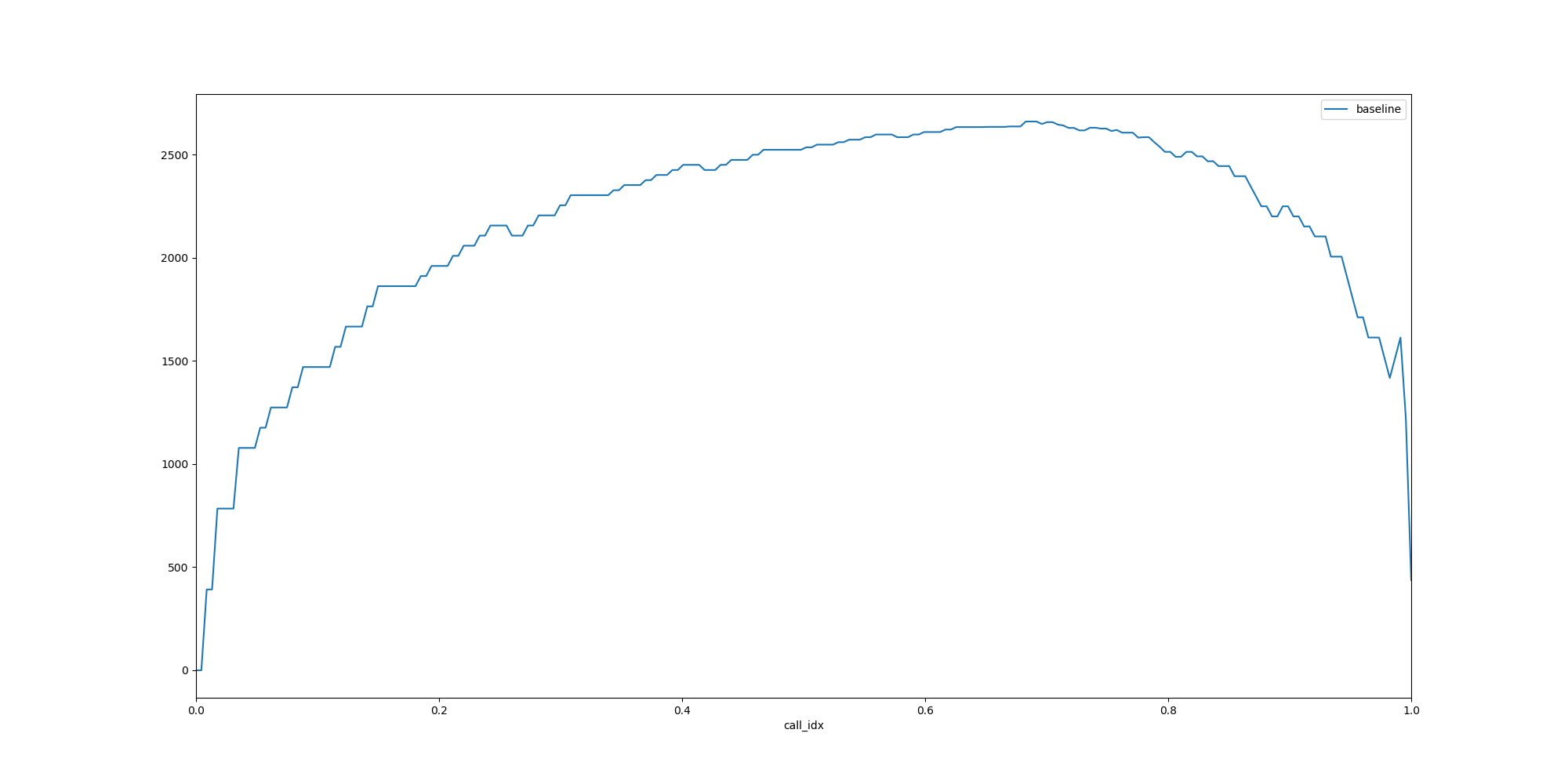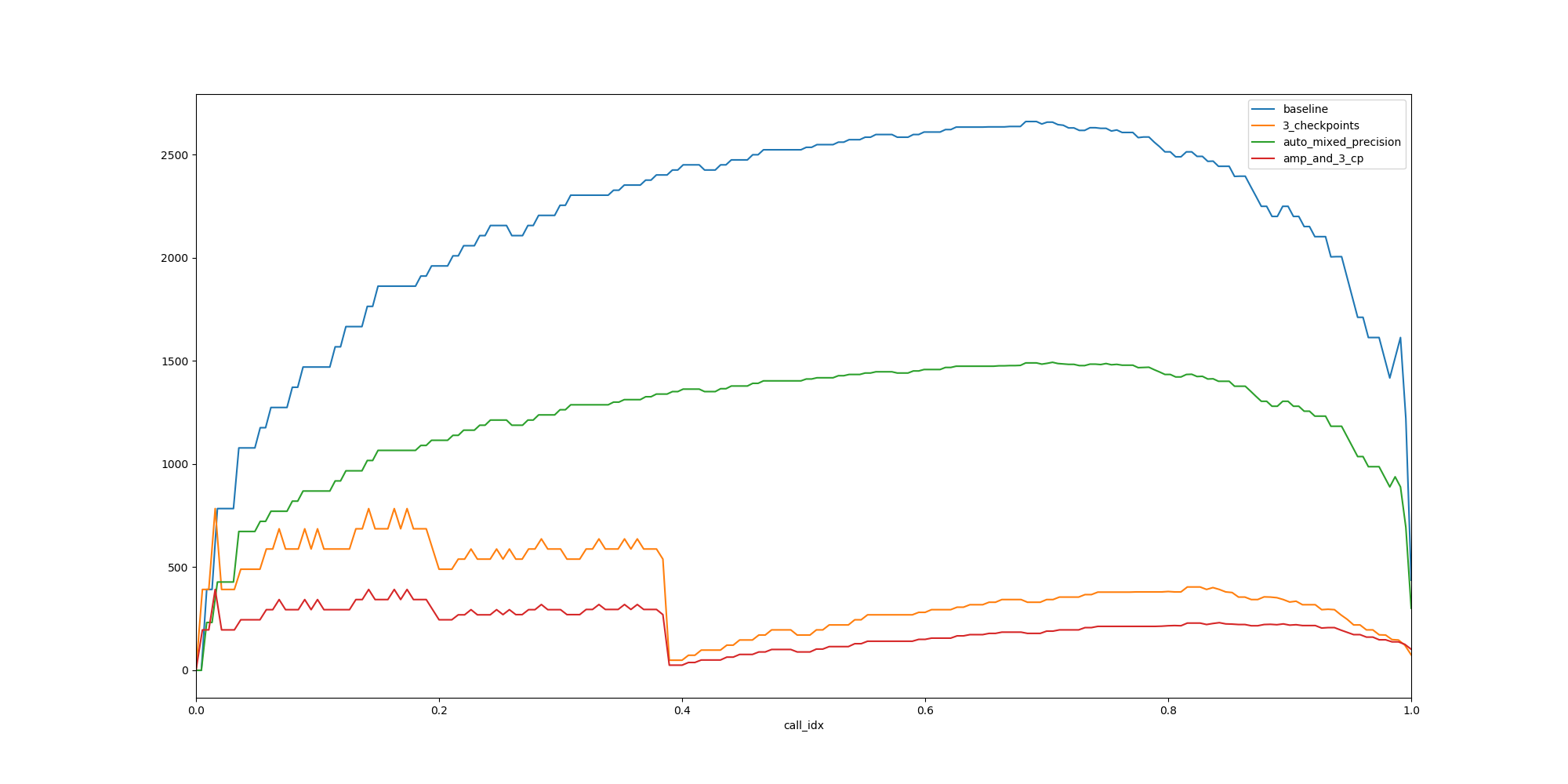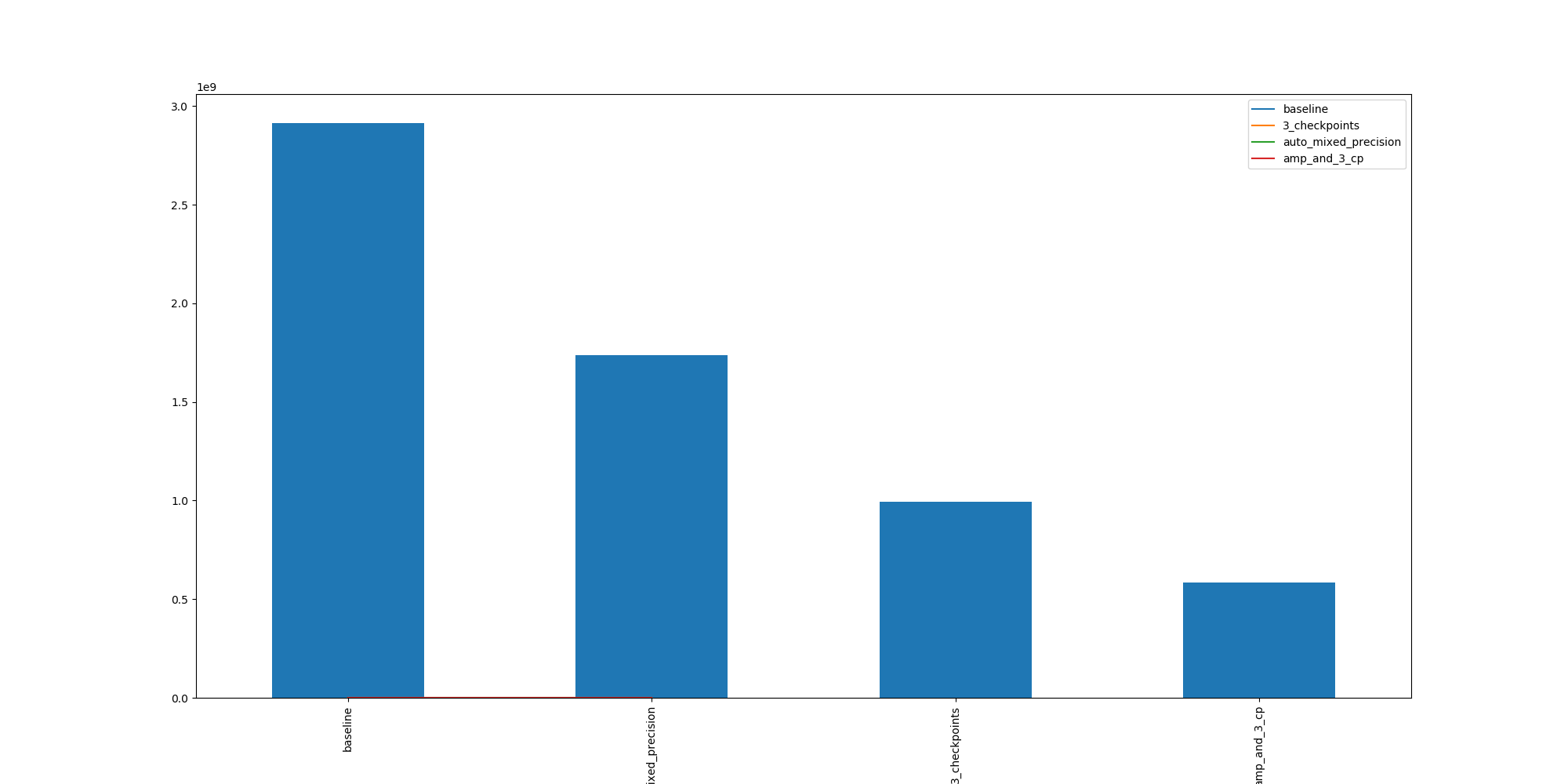# Deep Learning Memory Usage and Pytorch OptimizationShedding some light on the causes behind CUDA out of memory ERROR, and an example on how to reduce by 80% your memory footprint with a few lines of code in Pytorch

Shedding some light on the causes behind CUDA out of memory ERROR, and an example on how to reduce by 80% your memory footprint with a few lines of code in Pytorch

## Understanding memory usage in deep learning models training

In this first part, I will explain how a deep learning models that use a few hundred MB for its parameters can crash a GPU with more than 10GB of memory during their training !

So where does this need for memory comes from? Below I present the two main high-level reasons why a deep learning training need to store information:

• information necessary to backpropagate the error (gradients of the activation w.r.t. the loss)

• information necessary to compute the gradient of the model parameters

If there is one thing you should take out from this article, it is this:

As a rule of thumb, each layer with learnable parameters will need to store its input until the backward pass.

This means that every batchnorm, convolution, dense layer will store its input until it was able to compute the gradient of its parameters.

### Backpropagation of the gradients and the chain ruleNow even some layer without any learnable parameters need to store some data! This is because we need to backpropagate the error back to the input and we do this thanks to the chain rule:

Chain rule:(a_i being the activations of the layer i)

The culprit in this equation is the derivative of the input w.r.t the output. Depending on the layer, it will

• be dependent on the parameters of the layer (dense, convolution…)

• be dependent on nothing (sigmoid activation)

• be dependent on the values of the inputs:

eg MaxPool, ReLU …

For example, if we take a ReLU activation layer, the minimum information we need is the sign of the input.

Different implementations can look like:

• We store the whole input layer

• We store a binary mask of the signs (that takes less memory)

• We check if the output is stored by the next layer. If so, we get the sign info from there and we don’t need to store additional data

• Maybe some other smart optimization I haven’t thought of…

### Example with ResNet18

Now let’s take a closer look at a concrete example: The ResNet18!

We are going to look at the memory allocated on the GPU at specific times of the training iteration:

• At the beginning of the forward pass of each module

• At the end of the forward pass of each module

• At the end of the backward pass of each module

Full code and Github repo available here

The logger looks like this:

pytorch_log_mem.py

``````def _get_gpu_mem(synchronize=True, empty_cache=True):

def _generate_mem_hook(handle_ref, mem, idx, hook_type, exp):
def hook(self, *args):
if len(mem) == 0 or mem[-1]["exp"] != exp:
call_idx = 0
else:
call_idx = mem[-1]["call_idx"] + 1

mem_all, mem_cached = _get_gpu_mem()
torch.cuda.synchronize()
mem.append({
'layer_idx': idx,
'call_idx': call_idx,
'layer_type': type(self).__name__,
'exp': exp,
'hook_type': hook_type,
'mem_all': mem_all,
'mem_cached': mem_cached,
})

return hook

def _add_memory_hooks(idx, mod, mem_log, exp, hr):
h = mod.register_forward_pre_hook(_generate_mem_hook(hr, mem_log, idx, 'pre', exp))
hr.append(h)

h = mod.register_forward_hook(_generate_mem_hook(hr, mem_log, idx, 'fwd', exp))
hr.append(h)

h = mod.register_backward_hook(_generate_mem_hook(hr, mem_log, idx, 'bwd', exp))
hr.append(h)

def log_mem(model, inp, mem_log=None, exp=None):
mem_log = mem_log or []
exp = exp or f'exp_{len(mem_log)}'
hr = []
for idx, module in enumerate(model.modules()):

try:
out = model(inp)
loss = out.sum()
loss.backward()
finally:
[h.remove() for h in hr]

return mem_log``````

Then we can look at the memory consumption for the resnet18 (from the torchvision.models) with the following code:

log_resnet18_mem.py

``````# %% Analysis baseline

model = resnet18().cuda()
bs = 128
input = torch.rand(bs, 3, 224, 224).cuda()

mem_log = []

try:
mem_log.extend(log_mem(model, input, exp='baseline'))
except Exception as e:
print(f'log_mem failed because of {e}')

df = pd.DataFrame(mem_log)

plot_mem(df, exps=['baseline'], output_file=f'{base_dir}/baseline_memory_plot_{bs}.png')
``````Memory consumption during one training iteration of a ResNet18

A few things to observe:

• The memory keeps increasing during the forward pass and then starts decreasing during the backward pass

• The slope is pretty steep at the beginning and then flattens:

→ The activations become lighter and lighter when we go deeper into the network

• We have a maximum memory of about 2500 MB

Optional: the next section digs deeper into the shape of the plot

Let’s try to understand why memory usage is more important in the first layers.

For this, I display the memory impact in MB of each layer and analyse it.

• The indentation levels represent the parent/submodules relationship (e.g. the ResNet, is the root torch.nn.Module)

• On one line we see:

→The name of the Module

→The hook concerned. `pre`: before the forward pass,`fwd`: at the end of the forward pass, `bwd`: at the end of the backward pass)

→The GPU memory difference with the previous line, if there is any (in MegaBytes)

``````ResNet pre     # <-  shape of the input (128, 3, 224, 224)
Conv2d pre
Conv2d fwd  392.0  # <-  shape of the output (128, 64, 112, 112)
BatchNorm2d pre
BatchNorm2d fwd  392.0
ReLU pre
ReLU fwd
MaxPool2d pre
MaxPool2d fwd  294.0 # <- shape of the output (128, 64, 56, 56)
Sequential pre
BasicBlock pre
Conv2d pre
Conv2d fwd  98.0 # <-- (128, 64, 56, 56)
BatchNorm2d pre
BatchNorm2d fwd  98.0
ReLU pre
ReLU fwd
Conv2d pre
Conv2d fwd  98.0
BatchNorm2d pre
BatchNorm2d fwd  98.0
ReLU pre
ReLU fwd
BasicBlock fwd
...
...
ResNet fwd  # <-- End of the forward pass
Linear bwd  2.0 # <-- Beginning of the backward pass
...
...
BatchNorm2d bwd  -98.0
Conv2d bwd  -98.0
MaxPool2d bwd  98.0
ReLU bwd  98.0
BatchNorm2d bwd  -392.0
Conv2d bwd  -784.0 # <-- End of the backward pass
``````

### + 392 MB after the first convolution layer:

The input shape of the layer is :

• batch_size: 128

• input_channel: 3

• image dimensions: 224 x 224

The layer is :Conv2d(3, 64, kernel_size=(7, 7), stride=(2, 2), padding=(3, 3), bias=False)

The output shape of the layer is :

• batch_size: 128

• input_channel: 64

• image dimensions: 112 x 112

The additional allocation size for the output is:

(128 x 64 x 112 x 112 x 4) / 2**20 = 392 MB

(NB: the factor 4 comes from the storage of each number in 4 bytes as `FP32`, the division comes from the fact that 1 MB = 2**20 B)

Note also that this additional memory will not be freed once we moved on to the next layers

### + 98 MB after the second convolution layer:

Here we went through a max-pooling which divided the height and the width of the activations by 2.

The conv layer conserves the dimensions: Conv2d(64, 64, kernel_size=(3, 3), stride=(1, 1), padding=(1, 1), bias=False)

(128 x 64 x 56 x 56 x 4) / 2**20 = 98 MB (=392/4)

## Pytorch Optimization tricks on the shelf

Next, I will first present two ideas and their implementation in Pytorch to divide by 5 the footprint of the resnet in 4 lines of code :)

The idea behind gradient checkpointing is pretty simple:

If I need some data that I have computed once, I don’t need to store it: I can compute it again

So basically instead of storing all the layers’ inputs, I will store a few checkpoints along the way during the forward pass, and when I need some input that I haven’t stored I’ll just recompute it from the last checkpoint.

Plus it’s really easy to implement in Pytorch, especially if you have a nn.Sequential module. To apply it , I changed the line 9 of the log function as below:

log_mem_cp.py

``````def log_mem_cp(model, inp, mem_log=None, exp=None, cp_chunks=3):
mem_log = mem_log or []
exp = exp or f'exp_{len(mem_log)}'
hr = []
for idx, module in enumerate(model.modules()):

try:
out = checkpoint_sequential(model, cp_chunks, inp)
loss = out.sum()
loss.backward()
finally:
[h.remove() for h in hr]

return mem_log``````

And since it takes an instance of nn.Sequential, I created it as such

resnet_seq.py

``````# %% Create Sequential version of model
class Flatten(nn.Module):
def forward(self, x):

seq_model = nn.Sequential(
model.conv1,
model.bn1,
model.relu,
model.maxpool,
model.layer1,
model.layer2,
model.layer3,
model.layer4,
model.avgpool,
Flatten(),
model.fc,
)

# %% Test models are identical:

out = model(input)
seq_out = seq_model(input)
max_diff = (out - seq_out).max().abs().item()
assert max_diff < 10**-10``````

### Automatic mixed precision

The idea behind mixed-precision training is the following:

If we store every number on 2 bytes instead of 4: we’ll use half the memory

→But then the training doesn’t converge…

To fix this, different techniques are combined (loss scaling, master weight copy, casting to FP32 for some layers…).

The implementation of mixed-precision training can be subtle, and if you want to know more, I encourage you to go to visit the resources at the end of the article.

• Thankfully everything has been beautifully automatized in the Pytorch module!

So we can with only a couple of changes get some nice memory optimization (check lines 6, 7, 14, 15)

log_mem_amp.py

``````def log_mem_amp(model, inp, mem_log=None, exp=None):
mem_log = mem_log or []
exp = exp or f'exp_{len(mem_log)}'
hr = []
optimizer = torch.optim.SGD(model.parameters(), lr=0.01, momentum=0.9)
amp_model, optimizer = amp.initialize(model, optimizer)
for idx, module in enumerate(amp_model.modules()):

try:
out = amp_model(inp)
loss = out.sum()
with amp.scale_loss(loss, optimizer) as scaled_loss:
scaled_loss.backward()
finally:
[h.remove() for h in hr]

return mem_log``````

### Why not Both?

Then we can combine both into the following :

log_amp_cp.py

``````def log_mem_amp_cp(model, inp, mem_log=None, exp=None, cp_chunks=3):
mem_log = mem_log or []
exp = exp or f'exp_{len(mem_log)}'
hr = []
optimizer = torch.optim.SGD(model.parameters(), lr=0.01, momentum=0.9)
amp_model, optimizer = amp.initialize(model, optimizer)
for idx, module in enumerate(amp_model.modules()):

try:
out = checkpoint_sequential(amp_model, cp_chunks, inp)
loss = out.sum()
with amp.scale_loss(loss, optimizer) as scaled_loss:
scaled_loss.backward()
finally:
[h.remove() for h in hr]

return mem_log``````

## Results (Finally):Memory consumption comparison of the optimizations method with the baseline

Here are the main facts to observe:

• AMP: The overall shape is the same, but we use less memory

• Checkpointing : We can see that the model does not accumulate memory during the forward pass

Below are the maximum memory footprint of each iteration, and we can see how we divided the overall footprint of the baseline by 5.Maximum memory consumption for each training iteration

Full code and Github repo available here

## Conclusion and next steps :

Some notes on the results:

• We only looked at the memory savings

• To have a better comparison, we need to look at two additional metrics: training speed

and `training accuracy`

My intuition on this would be:

• Checkpointing is slower than the baseline and achieves the `same accuracy`

• `AMP` is `faster` than the baseline and achieves a`lower accuracy`

To be confirmed in the next episode …

## Basic Data Types in Python | Python Web Development For Beginners

In the programming world, Data types play an important role. Each Variable is stored in different data types and responsible for various functions. Python had two different objects, and They are mutable and immutable objects.

## How To Compare Tesla and Ford Company By Using Magic Methods in Python

Magic Methods are the special methods which gives us the ability to access built in syntactical features such as ‘<’, ‘>’, ‘==’, ‘+’ etc.. You must have worked with such methods without knowing them to be as magic methods. Magic methods can be identified with their names which start with __ and ends with __ like __init__, __call__, __str__ etc. These methods are also called Dunder Methods, because of their name starting and ending with Double Underscore (Dunder).

## Python Programming: A Beginner’s Guide

Python is an interpreted, high-level, powerful general-purpose programming language. You may ask, Python’s a snake right? and Why is this programming language named after it?

## Hire Python Developers

Are you looking for experienced, reliable, and qualified Python developers? If yes, you have reached the right place. At **[HourlyDeveloper.io](https://hourlydeveloper.io/ "HourlyDeveloper.io")**, our full-stack Python development services...

## Python any: How to Check If Element is Iterable or Not

Python any() function returns True if any element of an iterable is True otherwise any() function returns False. The syntax is any().